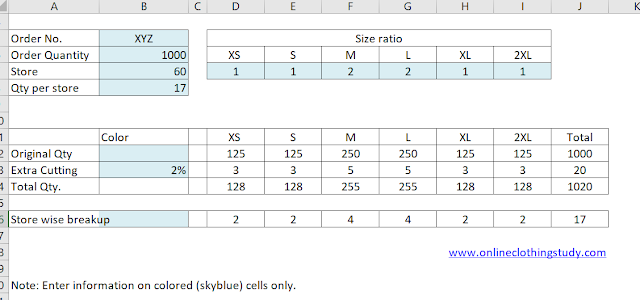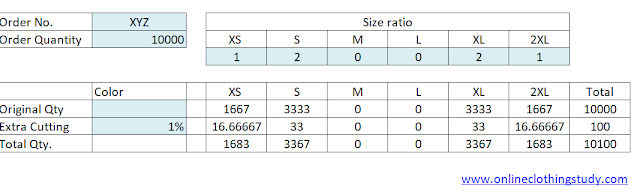# How to Calculate Size Wise Cut Quantity from the Size Ratio in an Order?

How to calculate size wise cutting quantity for 10000 pieces? For example, suppose we have received a production order of 10000 pieces and the order size ratio is S:M:L:XL = 1:2:2:1. Please help me with the formula. - asked by Mala SN

In bulk apparel production, garment manufacturers get an order in volume for a style. In such bulk orders, garment supplier needs to garment for multiple sizes. Like, the same design is made for size small, size medium and size Large.

The buyer PO contains total order quantity and size wise order quantity. Sometimes buyers do not provide the size wise quantity breakup. Instead, they provide the following details.
• total order quantity,
• list of sizes garment to made and
• size wise quantity ratio of garments
From the ratio, you need to calculate size wise quantity. A mathematical calculation is used to convert ratios into quantity.  At the time of cut order plan, size wise quantity is calculated by the garment manufacturers.

You can use the following steps to find size wise quantity.

Step#1. Calculate the sum of all ratios. Here size ratio (1:2:2:1), 1+2+2+1 =6

Step#2. Divide total order quantity by sum of the ratio (here 6)

Step#3. Multiply the result by the ratio of a particular size

The following formula is used for conversion from ratio to quantity.
Quantity of a size = (order quantity X ratio of the particular size)/ (Sum of ratio of all sizes)
Let's consider the above data for an example.  size wise cutting quantity will be

Quantity in S = (10000 /6) *1 = 1666.66 = 1667

Quantity in M = (10000 /6) *2 = 3333.33 = 3333

Quantity in L = (10000 /6) *2 = 3333.33 = 3333

Quantity in XL = (10000 /6) *1 = 1666.66 = 1667

The above calculation can be made easily by using an excel sheet. You can create a table with formula. Later when you get an order and size ratio, enter those on the specific cells; you will get actual cut quantity. For your reference, I have shown you a simple cut order plan (for a single color order) in the following table.Image#1: Cut order plan sheet for single color

If you find it difficult to calculate size wise cut quantity from the total order quantity using the given size ratio. Use my template.

### How to use this template?

• Enter your style number, order quantity, and the size ratio in the colored cells.
• If you like to add extra cutting to cover the defective garment and size mismatch in packing, add how extra quantity requirement in percentage. Like 3% or 5% or 7%.
• Once the entry is done in the colored cells, your size-wise quantity breakup is ready.

Question#2: Another question related to cut quantity break up from the ratio.
My question is if I want XS and  2XL size then how can we use this ratio of 1:2:2:1 can you
please guide me or make a formula sheet like that complete from XS to 2XL size. As our supply chain sent us demands like this. I have downloaded the template.

The formula is already there in the Excel template. You just need to enter the size ratio value in the cells (XS and 2XL).  In the image one (Image#1), you see no ratio is entered for size XS and 2XL.  That is why no quantity appeared in the size-wise quantity breakup matrix.

If you need to use all six sizes, in your order, you must have a size ratio for six sizes (e.g. 1:1:2:2:1:1). Please see the below image#2.Image#2: Using all sizes sizes.

If you need only four sizes out of these 6 sizes, then fill in the ratio in those sizes only. Let's say, your order has sizes like XS:S:L: XL=1:2:2:1. Enter these numbers in the template as per size.  You will get the quantity breakup for your order qty. Please refer to image#3.Image#3: 4 sizes

Question#3
If our company outlets are 60 then what is the planning calculator to send for example my 1000 product to all? This question belongs to the replenishment of the SKU for some sizes.

See the below example for distributing 1000 pieces into 60 stores (Image#4).
To match qty, you can send 16 pieces of garments to 20 stores and 17 pieces to 40 stores. You need to do that using manual adjustment.Image#4: Quantity break up by store

Related Question#4:
Can you please guide me that your Excel sheet 1:2:2:1  is about one colour distribution if we have multiple colors means the total order is 10000 but in 4 colors then what would we do?